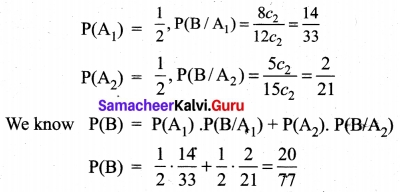# Tamil Nadu 11th Maths Model Question Paper 4 English Medium

Students can Download Tamil Nadu 11th Maths Model Question Paper 4 English Medium Pdf, Tamil Nadu 11th Maths Model Question Papers helps you to revise the complete Tamilnadu State Board New Syllabus and score more marks in your examinations.

## TN State Board 11th Maths Model Question Paper 4 English Medium

General Instructions:

1. The question paper comprises of four parts.
2. You are to attempt all the parts. An internal choice of questions is provided wherever applicable.
3. All questions of Part I, II, III and IV are to be attempted separately.
4. Question numbers 1 to 20 in Part I are Multiple Choice Questions of one mark each.
These are to be answered by choosing the most suitable answer from the given four alternatives and writing the option code and the corresponding answer
5. Question numbers 21 to 30 in Part II are two-mark questions. These are to be answered in about one or two sentences.
6. Question numbers 31 to 40 in Part III are three-mark questions. These are to be answered in above three to five short sentences.
7. Question numbers 41 to 47 in Part IV are five-mark questions. These are to be answered in detail Draw diagrams wherever necessary.

Time: 2.30 Hours
Maximum Marks: 90

PART – I

I. Choose the correct answer. Answer all the questions. [20 × 1 = 20]

Question 1.
The number of relations on a set containing 3 elements is………..
(a) 9
(b) 81
(c) 512
(d) 1024
Solution:
(c) 512

Question 2.
If n[(A × B) ∩ (A × C)] = 12 and n(B ∩ C) = 2 then n(A) is……….
(a) 2
(b) 3
(c) 4
(d) 6
Solution:
(d) 6Question 3.
If |x – 3| ≤ 5 then x belongs to………
(a) [-2, 8]
(b) (-2, 8)
(c) [-2, ∞]
(d) (-∞, 8)
Solution:
(a) [-2, 8]

Question 4.
The number of solutions of x² + |x – 1| = 1 is
(a) 1
(b) 0
(c) 2
(d) 3
Solution:
(c) 2

Question 5.
If a, 8, b are in A.P. a, 4, b are in G.P. and a, x, b are in H.P then x = ………..
(a) 2
(b) 1
(c) 4
(d) 16
Solution:
(a) 2

Question 6.
If 10 lines are drawn in a plane such that no two of them are parallel and no three are concurrent, then the total number of points of intersection are………..
(a) 45
(b) 40
(c) 10!
(d) 210
Solution:
(a) 45

Question 7.
The value of e2logx………..
(a) 2x
(b) x²
(c) √2
(d) $$\frac{x}{2}$$
Solution:
(b) x²

Question 8.
The nth term of the sequence 1, 2, 4, 7, 11 …. is ………..
(a) n³ + 3n² + 2n
(b) n³ – 3n² + 3n
(c) $$\frac{n(n+1)(n+2)}{3}$$
(d) $$\frac{n²-n+2}{2}$$
Solution:
(d) $$\frac{n²-n+2}{2}$$

Question 9.
The last term in the expansion (2 + √3)8 is
(a) 81
(b) 27
(c) 9
(d) 3
Solution:
(a) 81

Question 10.
A line perpendicular to the line 5x – y = 0 forms a triangle with the coordinate axes. If the area of the triangle is 5 sq.units, then its equation is………….
(a) x + 5y ± 5√2 = 0
(b) x – 5y ± 5√2 = 0
(c) 5x + y ± 5√2 = 0
(d) 5x – y ± 5√2 = 0
Solution:
(a) x + 5y ± 5√2 = 0

Question 11.
A factor of the determinant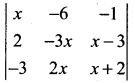is ……….
(a) x + 3
(b) 2x – 1
(c) x – 2
(d) x – 3
Solution:
(a) x + 3

Question 12.
If λ$$\vec {i}$$ + 2λ$$\vec {j}$$ + 2λ$$\vec {k}$$ is a unit vector then the value of λ is…………
(a) $$\frac{1}{3}$$
(b) $$\frac{1}{4}$$
(c) $$\frac{1}{9}$$
(d) $$\frac{1}{2}$$
Solution:
(a) $$\frac{1}{3}$$

Question 13.
One of the diagonals of parallelogram ABCD with $$\vec {a}$$ and $$\vec {b}$$ are adjacent sides is The other diagonal BD is…………
(a) $$\vec {a}$$ + $$\vec {b}$$.
(b) $$\vec {a}$$ – $$\vec {b}$$
(c) $$\vec {b}$$ – $$\vec {a}$$
(d) $$\frac{\vec a+\vec b}{2}$$
Solution:
(b) $$\vec {a}$$ – $$\vec {b}$$

Question 14.
If (1, 2, 4) and (2, -3λ, -3) are the initial and terminal points of the vector $$\vec {i}$$ + 5$$\vec {j}$$ – 7$$\vec {k}$$ then the value of λ ………….
(a) $$\frac{7}{3}$$
(b) –$$\frac{7}{3}$$
(c) $$\frac{5}{3}$$
(d) $$\frac{-5}{b}$$
Solution:
(b) –$$\frac{7}{3}$$Question 15.
If y = mx + c and f(0) = f'(0) = 1 then f(2) =…………
(a) 1
(b) 2
(c) 3
(d) 4
Solution:
(c) 3

Question 16.
The derivative of (x + $$\frac{1}{x}$$)² w.r.to. x is…………
(a) 2x – $$\frac{2}{x³}$$
(b) 2x + $$\frac{2}{x³}$$
(c) 2(x + $$\frac{1}{x}$$)
(d) 0
Solution:
(a) 2x – $$\frac{2}{x³}$$

Question 17.
If f(x) =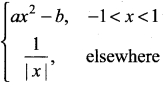is differentiable at x = 1, then………
(a) a = $$\frac{1}{2}$$, b = $$\frac{-3}{2}$$
(b) a = $$\frac{-1}{2}$$, b = $$\frac{3}{2}$$
(c) a = –$$\frac{1}{2}$$, b = –$$\frac{3}{2}$$
(d) a = $$\frac{1}{2}$$, b = $$\frac{3}{2}$$
Solution:
(c) a = –$$\frac{1}{2}$$, b = –$$\frac{3}{2}$$

Question 18.
∫sin 7x cos 5x dx =…………
(a) $$\frac{1}{2}$$ [$$\frac{cos 12x}{2}$$+$$\frac{cos 2x}{2}$$] + c
(b) –$$\frac{1}{2}$$ [$$\frac{cos 12x}{2}$$+$$\frac{cos 2x}{2}$$] + c
(c) –$$\frac{1}{2}$$ [$$\frac{cos 6x}{6}$$ + cos x] + c
(d) –$$\frac{1}{2}$$ [$$\frac{sin 12x}{2}$$+$$\frac{sin 2x}{2}$$] + c
Solution:
(b) –$$\frac{1}{2}$$ [$$\frac{cos 12x}{2}$$+$$\frac{cos 2x}{2}$$] + c

Question 19.
∫ $$\frac{1}{e^x}$$ dx = ………..
(a) log ex + c
(b) x + c
(c) $$\frac{1}{e^x}$$ + c
(d) $$\frac{-1}{e^x}$$
Solution:
(d) $$\frac{-1}{e^x}$$

Question 20.
Two items are chosen from a lot containing twelve items of which four are defective. Then the probability that atleast one of the item is defective is…………
(a) $$\frac{19}{33}$$
(b) $$\frac{17}{33}$$
(c) $$\frac{23}{33}$$
(d) $$\frac{13}{34}$$
Solution:
(a) $$\frac{19}{33}$$

PART – II

II. Answer any seven questions. Question No. 30 is compulsory. [7 × 2 = 14]

Question 21.
Prove that $$\frac{tanθ+secθ-1}{tanθ-secθ+1}$$ $$\frac{1+sinθ}{cosθ}$$
Solution: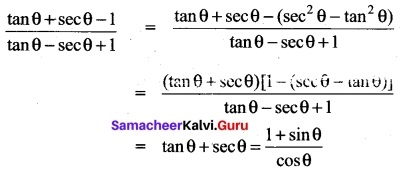Question 22.
Prove that the relation ‘friendship’ is not an equivalence relation on the set-of all people in chennai.
Solution:
S = aRa (i.e. ) a person can be a friend to himself or herself.
So it is reflextive.
aRb ⇒ bRa so it is symmetric
aRb, bRc does not ⇒ aRc
so it is not transitive
⇒ it is not an equivalence relation.

Question 23.
How many triangles can be formed by joining 15 points on the plane, in which no line joining any three points?
Solution:
No. of non-collinear points = 15
To draw a Triangle we need 3 points
∴Selecting 3 from 15 points can be done in 15C3 ways.
∴ No. of Triangle formed = 15C3
= $$\frac{15×14×13}{3×2×1}$$ = 455

Question 24.
Expand (2x + 3)5
Solution:
By taking a = 2x, b = 3 and n = 5 in the binomial expansion of (a + b)n
we get (2x + 3)5 = (2x)5 + 5(2x)43 + 10(2x)³3² + 10(2x)²3³ + 5(2x)34 + 35
= 32x5 + 240x4 + 720x³ + 1980x² + 810x + 243.

Question 25.
If λ = -2, determine the value of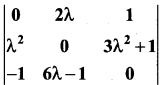Solution:
Given λ = -2
2λ = -4; λ² = (-2)² = 4; 3λ² + 1 = 3(4) + 1 = 13
6λ – 1 = 6(-2) – 1 = -13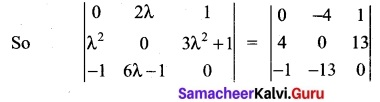expanding along R,
0(0) + 4 (0 + 13) +1 (-52 + 0) = 52 – 52 = 0
Aliter: The determinant value of a skew symmetric matrix is zero.

Question 26.
Compute $$\lim _{x \rightarrow 1} \frac{\sqrt{x}-1}{x-1}$$
Solution:
Here $$\lim _{x \rightarrow 1}$$(x – 1) = 0. In such cases, rationalise the numerator.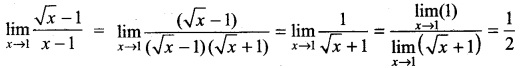Question 27.
Differentiate the following $$\frac{x²}{a²}$$+ $$\frac{y²}{b²}$$ = 1
Solution:
Given $$\frac{x²}{a²}$$+ $$\frac{y²}{b²}$$ = 1
Differentiating w.r.to x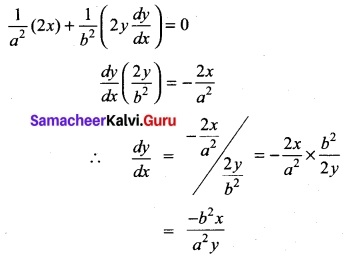Question 28.
Evaluate $$\frac{1}{(x+1)²-25}$$
Solution: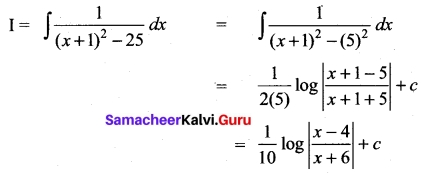Question 29.
Given that P(A) = 0.52, P(B) = 0.43, and P(A ∩ B) = 0.24, find p(A ∩ $$\bar { B }$$)
Solution:
P(A ∩ $$\bar { B }$$) = P(A) – P(A ∩ B)
= 0.52 – 0.24 = 0.28
P(A ∩ $$\bar { B }$$) = 0.28

Question 30.
Show that 4x² + 4xy + y² – 6x – 3y – 4 = 0 represents a pair of parallel lines
Solution:
4x² + 4xy + y² – 6x – 3y – 4 = 0
a = 4, b = 1, h = 4/2 = 2
h² – ab = 2² – (4) (1) = 4 – 4 = 0
⇒ The given equation represents a pair of parallel lines.

PART-III

III. Answer any seven questions. Question No. 40 is compulsory. [7 × 3 = 21]

Question 31.
If A and B are two sets so that n(B – A) = 2n(A – B) = 4n(A ∩ B) and if n(A ∪ B) = 14 then find n(PA)
Solution:
To find n(P(A)), we need n(A).
Let n( A ∩ B) = t. Then n( A – B) = 2k and n(B – A) = 4k.
Now n(A ∪ B) = n(A – B) + n(B – A) + n(A ∩ B) = 7k.
It is given that n(A ∪ B) = 14. Thus 7k = 14 and hence k = 2.
So n(A – B) = 4 and n(B – A) = 8. As n(A) = n(A – B) + n(A ∩ B), we get n(A) = 6 and hence n(P(A)) = 26 = 64.

Question 32.
Resolve $$\frac{1}{x²-a²}$$ into partial fraction.
Solution:
Factorizing the denominator
Dr = x² – a² = (x – a) (x + a)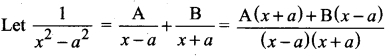Equating the numerator we get
x – a = 0
⇒ x = a
x + a = 0
⇒ x = -a
1 = A (x + a) + B (x – a)
This equation is true for any value of x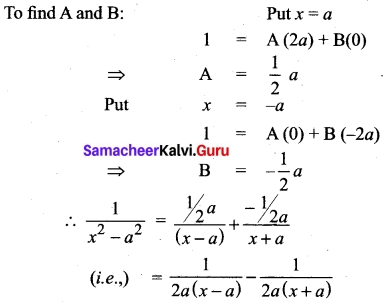Question 33.
Count the number of positive integers greater than 7000 and less than 8000 which are divisible by 5 provided that no digits are repeated.
Solution:
It should be a 4-digit number greater than 7000 and less than 8000. Then the thousand’s place will be the digit 7. Further, as the number must be divisible by 5 the unit place should be either 0 or 5.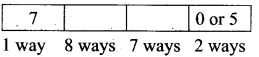As repetition is not permitted, the 100th place can be filled in 8 ways using remaining numbers and 10th place can be filled in 7 ways. Hence, the required number of numbers is 1 × 8 × 7 × 2 = 112.

Question 34.
Find the $$\sqrt{126}$$ approximately to two decimal places.
Solution: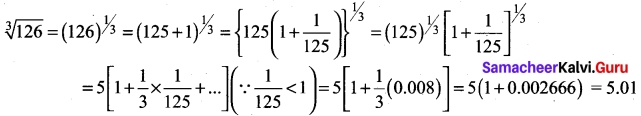Question 35.
Find the equation of the line through the intersection of the lines
3x + 2y + 5 = 0 and 3x – 4y + 6 = 0 and the point (1, 1)
Solution:
The family of equations of straight lines through the point of intersection of the lines is of the form (a1x + b1y + c1) + (a2x + b2y + c2) = 0
That is, (3x + 2y + 5) + λ (3x – 4y + 6) = 0
Since the required equation passes through the point (1, 1), the point satisfies the above equation Therefore {3 + 2(1) + 5} + λ(3 (1) – 4(1) + 6} = 0 ⇒ λ = -2
Substituting λ = -2 in the above equation we get the required equation as 3x – 10y + 7 = 0 (verify the above problem by using two points form)

Question 36.
Show that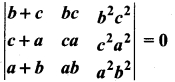Solution: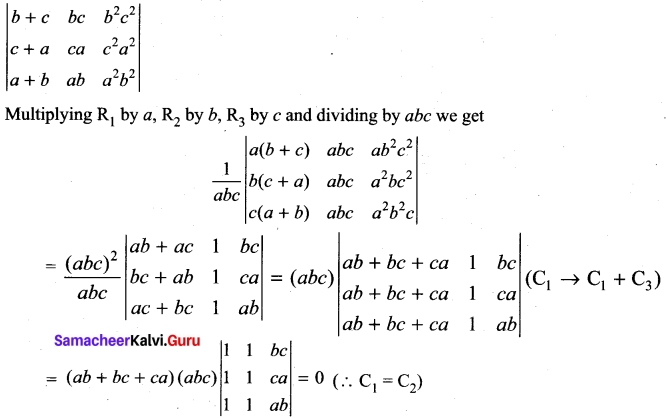Question 37.
Complete the following table using calculator and use the result to estimate $$\lim _{x \rightarrow 2}$$ $$\frac{x-2}{x²-x-2}$$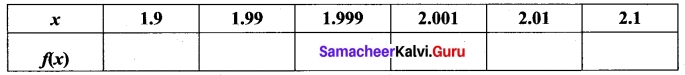Solution: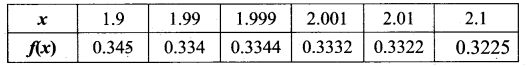Limit is 0.333…. = 0.$$\bar{3}$$Question 38.
Differentiate $$\frac{e^{3x}}{1+e^x}$$ with respect to x
Solution: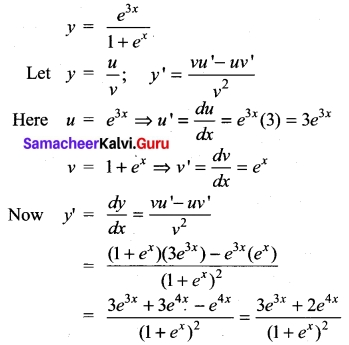Question 39.
Evaluate: ex (tan x + log sec x)
Solution:
Let I = ∫ex (tan x + log sec x) dx
Take f(x) = log sec x
f(x) = $$\frac{1}{sec x}$$ × sec x tan x = tan x
This is of the form ∫ex[f(x) + f'(x)] dx = ex f(x) + c
∴ ∫ex(log sec x + tan x) dx = ex log |sec x| + c

Question 40.
The position vectors of the vertices of a triangle are $$\vec{i}$$ + 2$$\vec{j}$$ + 3$$\vec{k}$$, 3$$\vec{i}$$ – 4$$\vec{j}$$ + 5$$\vec{k}$$ and -2$$\vec{i}$$ + 3$$\vec{j}$$ – 7$$\vec{k}$$ Find the perimeter of a triangle.
Solution:
Let A, B, C be the vertices of triangle ABC,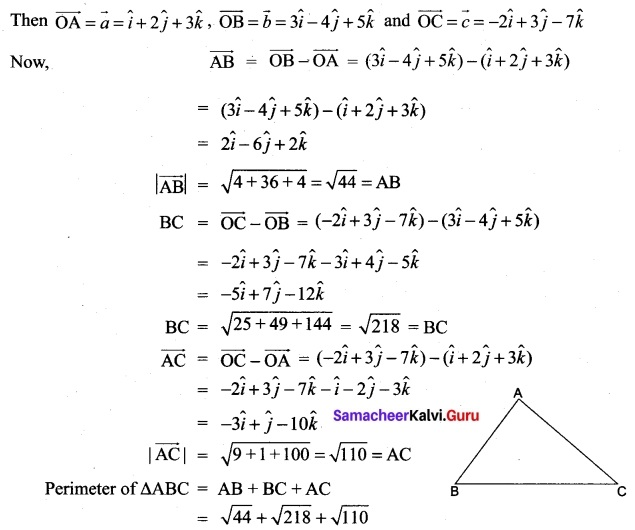PART – IV

IV. Answer all the questions. [7 × 5 = 35]

Question 41 (a).
If f : R → R is defined by f(x) = 3x – 5, prove that f is a bijection and find its inverse.
Solution:
p(x) = 3x – 5
Let g(y) = 3x – 5 ⇒ 3x = y + 5
x = $$\frac{y+5}{3}$$
Let g(y) = $$\frac{y+5}{3}$$
Now g o f(y) = g[(f(x))] = g(3x-5)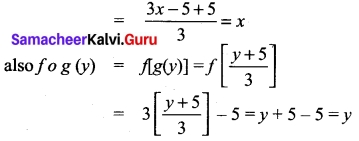Thus g o f = Ix and f o g = Iy
f and g are bi-jections and inverse to each other. Hence f is a bi-jection and f-1(y) = $$\frac{y+5}{3}$$
Replacing y by x we get f-1(x) = $$\frac{x+5}{3}$$[OR]

(b) Prove that tan-1($$\frac{m}{n}$$) – tan-1($$\frac{m-n}{m+n}$$) = $$\frac{π}{4}$$
Solution: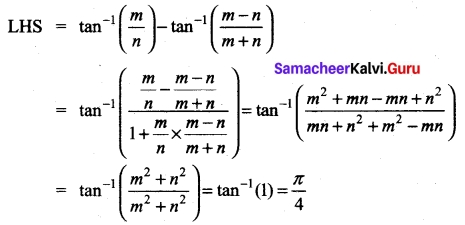Question 42 (a).
Find the values of k so that the equation x² = 2x (1 + 3k) + 7(3 + 2k) = 0 has real and equal roots.
Solution:
The equation is x² – x(2) (1 + 3k) – 7 (3 + 2k) = 0
The roots are real and equal
⇒ Δ = 0 (i.e.,) b² – 4ac = 0
Here a = 1, b = -2 (1 + 3k), c = 7(3 + 2k)
So b² – 4ac = 0 ⇒ [-2 (1 + 3k)]² – 4(1) (7) (3 + 2k) = 0
(i.e.,) 4(1+ 3k)² – 28 (3 + 2k) = 0
(÷ by 4) (1 + 3k)² – 7(3 + 2k) = 0
1 + 9k² + 6k – 21 – 14k = 0
9k² – 8k – 20 = 0
(k – 2) (9k + 10) = 0
⇒ k – 2 > 0 or 9k + 10 = 0
⇒ k = 2 or k = $$\frac{-10}{9}$$
To solve the quadratic inequalities ax² + bx + c < 0 (or) ax² + bx + c > 0

[OR]

(b) If the roots of the equation (q – r) x² + (r – p)x + (p – q) = 0 are equal then show that p, q and r are in A.P.
Solution:
The roots are equal ⇒ Δ = 0
(i.e.) b² – 4ac = 0
Hence, a = q – r; b = r – p; c = p – q
b² – 4ac = 0
⇒ (r – p)² – 4(q – r)(p – q) = 0
r² + p² – 2pr – 4[qr – q² – pr + pq] = 0
r² + p² – 2pr – 4qr +4q² + 4pr – 4pq = 0
(i.e.) p² + 4q² + r² – 4pq – 4qr + 2pr = 0
(i.e.) (p – 2q + r)² = 0
⇒ p – 2q + r = 0
⇒ p + r = 2q
⇒ p, q, r are in A.P.

Question 43 (a).
Find the sum of all 4 digit-numbers that can be formed using the digits 1, 2, 3, 4 and 5 repetition not allowed?
Solution:
The given digits are 1, 2, 3, 4, 5
The no. of 4 digit numbers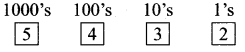= 5 × 4 × 3 × 2 = 120
(i.e) 5P4 = 120
Now we have 120 numbers
So each digit occurs $$\frac{120}{5}$$ = 24 times
Sum of the digits =1+2 + 3 + 4 + 5 = 15
Sum of number’s in each place = 24 × 15 = 360
Sum of numbers = 360 × 1 = 360
360 × 10 = 3600
360 × 100 = 36000
360 × 1000 = 360000
Total = 399960[OR]

(b) Three vectors $$\vec{a}$$, $$\vec{b}$$ and $$\vec{c}$$ are such that |$$\vec{a}$$| = 2,|$$\vec{b}$$| = 3, |$$\vec{c}$$| = 4 and $$\vec{a}$$ + $$\vec{b}$$ + $$\vec{c}$$ = 0. Find 4$$\vec{a}$$.$$\vec{b}$$ + 3$$\vec{b}$$.$$\vec{c}$$ + 3$$\vec{c}$$.$$\vec{a}$$.
Solution:
Given $$\vec{a}$$ + $$\vec{b}$$ + $$\vec{c}$$ = 0
⇒ $$\vec{a}$$ + $$\vec{a}$$ = –$$\vec{a}$$
so ($$\vec{a}$$ + $$\vec{a}$$)² = $$\vec{c}$$²
(i.e.) a² + b²+ 2$$\vec{a}$$.$$\vec{b}$$ = $$\vec{c}$$²
⇒ 4 + 9 + 2$$\vec{a}$$.$$\vec{b}$$ = 16
⇒ 2$$\vec{a}$$.$$\vec{b}$$ = 16 – 4 – 9 = 3
$$\vec{a}$$ $$\vec{b}$$ = 3/2
Again $$\vec{a}$$ + $$\vec{b}$$ + $$\vec{c}$$ = 0
⇒ $$\vec{a}$$ + $$\vec{c}$$ = –$$\vec{b}$$
($$\vec{a}$$ + $$\vec{c}$$)² = $$\vec{b}$$²
$$\vec{a}$$² + $$\vec{c}$$² + 2$$\vec{a}$$.$$\vec{c}$$ = $$\vec{b}$$²
4 + 16 + 2$$\vec{a}$$ – $$\vec{a}$$ = 9
2$$\vec{a}$$ – $$\vec{c}$$ = 9 – 4 – 16 = -11
$$\vec{a}$$.$$\vec{c}$$ = $$\frac{-11}{2}$$ (i.e.,) $$\vec{c}$$ – $$\vec{a}$$ = $$\frac{-11}{2}$$ (∵$$\vec{a}$$.$$\vec{c}$$ = $$\vec{c}$$.$$\vec{a}$$)
Also $$\vec{a}$$ + $$\vec{b}$$ + $$\vec{c}$$ = 0
$$\vec{b}$$ + $$\vec{c}$$ = –$$\vec{a}$$
($$\vec{b}$$ + $$\vec{c}$$)² = $$\vec{a}$$²
9 + 16 + 2$$\vec{b}$$ – $$\vec{c}$$ = 4
2$$\vec{b}$$ – $$\vec{c}$$ = 4 – 9 – 16 = -21
$$\vec{b}$$ – $$\vec{c}$$ = $$\frac{21}{2}$$
Here $$\vec{a}$$.$$\vec{b}$$ = 3/2 $$\vec{b}$$.$$\vec{c}$$ = $$\frac{-21}{2}$$ and $$\vec{c}$$.$$\vec{a}$$ = $$\frac{-11}{2}$$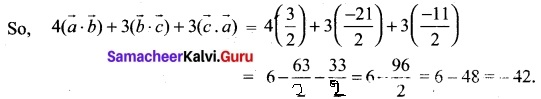Question 44 (a).
If a, b, c are respectively the pth, qth and rth terms of a G.P. show that (q – r) log a+ (r – p) log b + (p – q) log c = 0.
Solution:
Let the G.P. be l, lk, lk²,…
We are given tp = a, tq = b, tr = c
⇒ a = lkp-1; b = l kq-1; c = l kr-1
a = lkp-1 ⇒ log a = log l + log kp-1 = log l + (p – 1) log k
b = lkq-1 ⇒ log b = log l + log kq-1 = log l + (q – 1) log k
c = lkr-1 ⇒ log r = log l + log kr-1 = log l + ( r – 1) log k
LHS = (q – r) log a + (r – p) log b + (p – q) log c
= (q – r) [log l + (p – 1) log k ] + (r – p) [log l + (q – 1) log k]
(p – q) [log l + (r – 1) log k]
= log l[p – q + q – r + r – p] + log k[(q – r)(p – 1) + (r – p) (q – 1) + (p – q)(r – 1)]
= log l (0) + log k[p (q – r) + q (r – p) + r(p – q) – (q – r + r – p + p – q)]
= 0 = RHS.[OR]

(b) If A = $$\left[\begin{array}{ll} \frac{1}{2} & \alpha \\ 0 & \frac{1}{2} \end{array}\right]$$, Prove that $$\sum_{k=1}^{n}$$ det(Ak) = $$\frac{1}{3}$$ (1 – $$\frac{1}{4^n}$$)
Solution: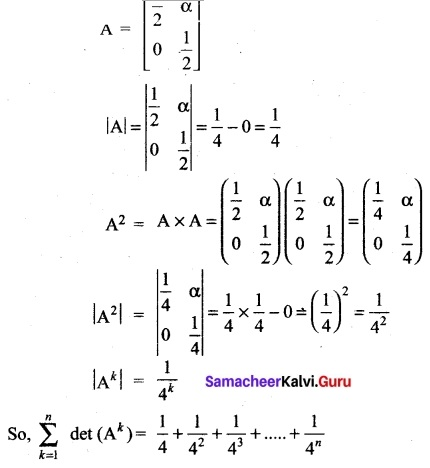Which is a G.P with a = $$\frac{1}{4}$$ and r = $$\frac{1}{4}$$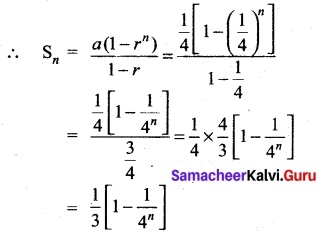Question 45 (a).
Find the equation of the straight line passing through intersection of the straight lines 5x – 6y = 1 and 3x + 2y + 5 = 0 and perpendicular to the straight line 3x – 5y + 11 = 0.
Solution:
Equation of line through the intersection of straight lines 5x – 6y = 1 and 3x + 2y + 5 = 0 is 5x – 6y – 1 + k (3x + 2y + 5) = 0 x(5 + 3k) + y(-6 + 2k) + (-1 + 5k) = 0
This is perpendicular to 3x – 5y + 11 = 0
That is, the product of their slopes is -1
–$$\frac{5+3k}{-6+2k}$$ (-$$\frac{3}{-5}$$) = -1
⇒ $$\frac{15+9k}{-30+10k}$$ = 1
⇒ 15 + 9k = -30 + 10k
45 = k
Required equation is 5x – 6y – 1 + 45 (3x + 2y + 5) = 0
140x + 84y + 224 = 0
20x + 12y + 32 = 0
5x + 3y + 8 = 0

[OR]

(b) Integrate the following $$\frac{√x}{1+√x}$$ dx
Solution: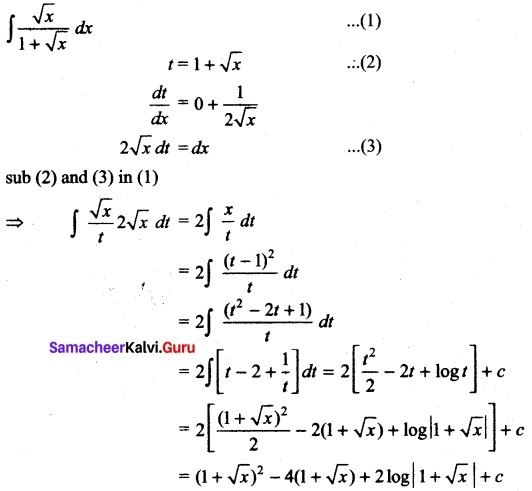Question 46 (a).
If u = tan-1($$\frac{\sqrt{1+x^{2}}-1}{x}$$) and v = tan-1x, find $$\frac{dx}{dy}$$
Solution: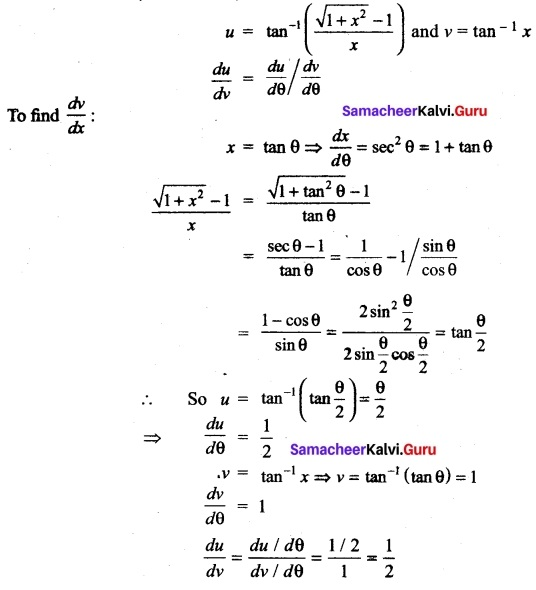[OR]

(b)
If y = Ae6x + Be-x prove that $$\frac{d²y}{dx²}$$ – 5$$\frac{dx}{dy}$$ – 6y = 0
Solution:
y = Ae6x + Be-x …. (1)
y1 = $$\frac{dx}{dy}$$ = Ae6x(6) + Be-x (-1)
= 6Ae6x – Be-x…. (2)
y2 = $$\frac{dx}{dy}$$ = 6Ae6x (6) – Be-x (-1)
= 36Ae6x + Be-x ……(3)
eliminating A and B from (1), (2) and (3) we get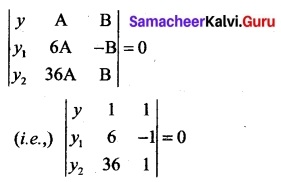y (6 + 36) – y1 (1 – 36) + y2 (-1 – 6) = 0
42y + 35y1 – 7y2 = 0
(÷ by -7) y2 – 5y1 – 6y = 0
(i.e.,) $$\frac{d²y}{dx²}$$ – 5$$\frac{dx}{dy}$$ – 6y = 0Question 47 (a).
Evaluate $$\lim _{x \rightarrow 0} \frac{\sqrt{x^{2}+1}-1}{\sqrt{x^{2}+16}-4}$$
Solution: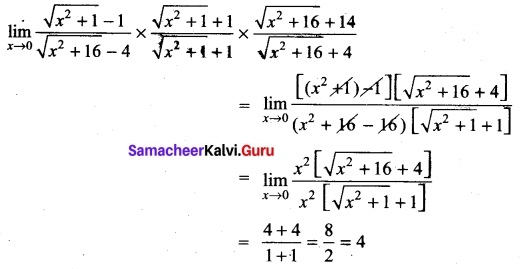[OR]
(b) Urn-I contains 8 red and 4 blue balls and urn-II contains 5 red and 10 blue balls. One urn is chosen at random and two balls are drawn from it. Find the probability that both balls are red.
Solution:
Let A1 be the event of selecting um-I and A2 be the event of selecting um-II.
Let B be the event of selecting 2 red balls.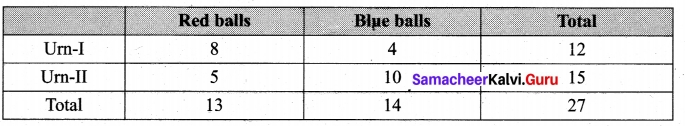We have to find the total probability of event B. That is, P(B).
Clearly A1 and A2A1 are mutually exclusive and exhaustive events.
We have